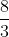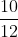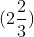Scan QR code or get instant email to install app

Question:

# 1212, 2.28,, 0.199. What number in the list above has the greatest value?

A 1212explanation

1212The fractionis greater than 1 because the numerator is larger than the denominator. This makes it greater thanand 0.199, which are both less than 1. The fractioncan be converted to a mixed numberor decimal (approximately 2.66) by dividing 8 by 3. The other choices are incorrect because(or approximately 2.66) is greater than 2.28,, and 0.199.